Next: The Initial value of Up: Initial Conditions on the Previous: Standing Waves: Preserving Isotropy

## Initial Conditions in One and Two Dimensions

To properly solve field theory in a one or two dimensional universe it would be necessary to quantize your fields in this space and derive the expressions for the mode amplitudes and other quantities there. In practice, however, this is not the problem that LATTICEEASY is intended to solve. We are interested in the evolution of fields in a three dimensional space, and the option to do these simulations in lower dimensions is intended to be viewed as a computationally convenient way of solving that same problem. Thus our intention in setting the initial conditions for the modes in these lower dimensions is to duplicate as accurately as possible the field values on a slice through a three dimensional space.

In practice, we approximate such a result by initializing our modes in such as a way as to achieve the same initial value of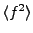as we would in a three dimensional simulation. To see how this works we denote the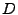dimensional Fourier transform of the field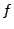as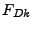. Parseval's theorem for a discrete Fourier transform in three dimensions says(6.79)

Averaging over the lattice in position space gives(6.80)

Given that the field distribution in Fourier space is on average isotropic this sum can be approximated as a one dimensional sum(6.81)

where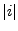is the distance from the origin of the lattice measured in units of the grid spacing. Thearises from the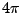that comes from three dimensional integration of an isotropic function, divided by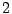to account for the fact that the one dimensional sum is taken over both positive and negative. Recall from section 6.3.2 that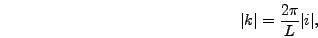(6.82)

so(6.83)

Comparing this result to Parseval's theorem in one dimension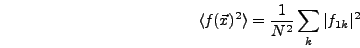(6.84)

gives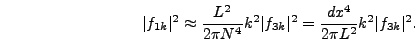(6.85)

Similar logic gives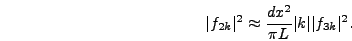(6.86)

We initialize the modes in one or two dimensions by using the formulas derived above for three dimensions and then adjusting each mode by the factor derived here. Note that these approximations are somewhat inaccurate because the space is cubical rather than spherical. This could be corrected by introducing an extra constant factor to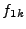andbut in practice we haven't found this difference to be relevant.Next: The Initial value of Up: Initial Conditions on the Previous: Standing Waves: Preserving Isotropy